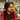# BST Post Order Traversal

BST Construction is covered here.

Sample tree:

``````           5
/     \
2       9
/     \      \
1     3       10``````

## Post Order traversal:

The first element will be the left child, then the right, then the root element at the last.

`[1, 3, 2, 10, 9, 5]`

## Recursive solution:

``````var traversal = []

function preorder(root) {
if (root == null) return

preorder(root.left)
preorder(root.right)
traversal.push(root.val)
}``````

## Iterative solution:

Depth first search, using a stack.

``````var traversal = []
var stack = []

while (stack.length != 0 || root != null) {
if (root) {
stack.push(root)
root = root.left
} else {
var temp = stack[stack.length - 1]
if (temp.right) {
root = temp.right
} else {
temp = stack.pop()
res.push(temp.val)

while (stack.length != 0 && stack[stack.length - 1].right == temp) {
temp = stack.pop()
res.push(temp.val)
}
}
}
}``````

## Other BST traversals:Written by Gagandeep Rangi who likes to talk about himself in third person. Twitter Instagram